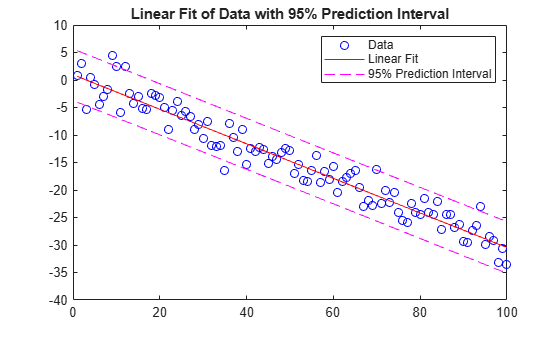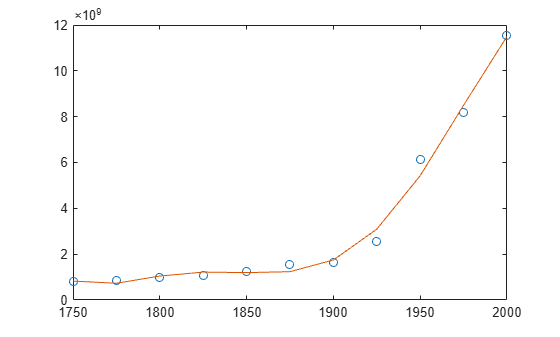# polyval

Polynomial evaluation

## Syntax

``y = polyval(p,x)``
``[y,delta] = polyval(p,x,S)``
``y = polyval(p,x,[],mu)``
``````[y,delta] = polyval(p,x,S,mu)``````

## Description

example

````y = polyval(p,x)` evaluates the polynomial `p` at each point in `x`. The argument `p` is a vector of length `n+1` whose elements are the coefficients (in descending powers) of an `n`th-degree polynomial:$p\left(x\right)={p}_{1}{x}^{n}+{p}_{2}{x}^{n-1}+...+{p}_{n}x+{p}_{n+1}.$The polynomial coefficients in `p` can be calculated for different purposes by functions like `polyint`, `polyder`, and `polyfit`, but you can specify any vector for the coefficients.To evaluate a polynomial in a matrix sense, use `polyvalm` instead.```

example

````[y,delta] = polyval(p,x,S)` uses the optional output structure `S` produced by `polyfit` to generate error estimates. `delta` is an estimate of the standard error in predicting a future observation at `x` by `p(x)`.```

example

````y = polyval(p,x,[],mu)` or ```[y,delta] = polyval(p,x,S,mu)``` use the optional output `mu` produced by `polyfit` to center and scale the data. `mu(1)` is `mean(x)`, and `mu(2)` is `std(x)`. Using these values, `polyval` centers `x` at zero and scales it to have unit standard deviation,$\stackrel{^}{x}=\frac{x-\overline{x}}{{\sigma }_{x}}\text{\hspace{0.17em}}.$This centering and scaling transformation improves the numerical properties of the polynomial.```

## Examples

collapse all

Evaluate the polynomial $\mathit{p}\left(\mathit{x}\right)=3{\mathit{x}}^{2}+2\mathit{x}+1$ at the points $\mathit{x}=5,7,9$. The polynomial coefficients can be represented by the vector `[3 2 1]`.

```p = [3 2 1]; x = [5 7 9]; y = polyval(p,x)```
```y = 1×3 86 162 262 ```

Evaluate the definite integral

`$I={\int }_{-1}^{3}\left(3{x}^{4}-4{x}^{2}+10x-25\right)dx.$`

Create a vector to represent the polynomial integrand $3{x}^{4}-4{x}^{2}+10x-25$. The ${\mathit{x}}^{3}$ term is absent and thus has a coefficient of 0.

`p = [3 0 -4 10 -25];`

Use `polyint` to integrate the polynomial using a constant of integration equal to `0`.

`q = polyint(p)`
```q = 1×6 0.6000 0 -1.3333 5.0000 -25.0000 0 ```

Find the value of the integral by evaluating `q` at the limits of integration.

```a = -1; b = 3; I = diff(polyval(q,[a b]))```
```I = 49.0667 ```

Fit a linear model to a set of data points and plot the results, including an estimate of a 95% prediction interval.

Create a few vectors of sample data points (x,y). Use `polyfit` to fit a first degree polynomial to the data. Specify two outputs to return the coefficients for the linear fit as well as the error estimation structure.

```x = 1:100; y = -0.3*x + 2*randn(1,100); [p,S] = polyfit(x,y,1); ```

Evaluate the first-degree polynomial fit in `p` at the points in `x`. Specify the error estimation structure as the third input so that `polyval` calculates an estimate of the standard error. The standard error estimate is returned in `delta`.

`[y_fit,delta] = polyval(p,x,S);`

Plot the original data, linear fit, and 95% prediction interval $\mathit{y}±2\Delta$.

```plot(x,y,'bo') hold on plot(x,y_fit,'r-') plot(x,y_fit+2*delta,'m--',x,y_fit-2*delta,'m--') title('Linear Fit of Data with 95% Prediction Interval') legend('Data','Linear Fit','95% Prediction Interval')```Create a table of population data for the years 1750 - 2000 and plot the data points.

```year = (1750:25:2000)'; pop = 1e6*[791 856 978 1050 1262 1544 1650 2532 6122 8170 11560]'; T = table(year, pop)```
```T=11×2 table year pop ____ _________ 1750 7.91e+08 1775 8.56e+08 1800 9.78e+08 1825 1.05e+09 1850 1.262e+09 1875 1.544e+09 1900 1.65e+09 1925 2.532e+09 1950 6.122e+09 1975 8.17e+09 2000 1.156e+10 ```
`plot(year,pop,'o')`Use `polyfit` with three outputs to fit a 5th-degree polynomial using centering and scaling, which improves the numerical properties of the problem. `polyfit` centers the data in `year` at 0 and scales it to have a standard deviation of 1, which avoids an ill-conditioned Vandermonde matrix in the fit calculation.

`[p,~,mu] = polyfit(T.year, T.pop, 5);`

Use `polyval` with four inputs to evaluate `p` with the scaled years, `(year-mu(1))/mu(2)`. Plot the results against the original years.

```f = polyval(p,year,[],mu); hold on plot(year,f) hold off```## Input Arguments

collapse all

Polynomial coefficients, specified as a vector. For example, the vector `[1 0 1]` represents the polynomial ${x}^{2}+1$, and the vector `[3.13 -2.21 5.99]` represents the polynomial $3.13{x}^{2}-2.21x+5.99$.

Data Types: `single` | `double`
Complex Number Support: Yes

Query points, specified as a vector. `polyval` evaluates the polynomial `p` at the points in `x` and returns the corresponding function values in `y`.

Data Types: `single` | `double`
Complex Number Support: Yes

Error estimation structure. This structure is an optional output from `[p,S] = polyfit(x,y,n)` that can be used to obtain error estimates. `S` contains the following fields:

FieldDescription
`R`Triangular factor from a QR decomposition of the Vandermonde matrix of `x`
`df`Degrees of freedom
`normr`Norm of the residuals

If the data in `y` is random, then an estimate of the covariance matrix of `p` is `(Rinv*Rinv')*normr^2/df`, where `Rinv` is the inverse of `R`.

Centering and scaling values, specified as a two-element vector. This vector is an optional output from ```[p,S,mu] = polyfit(x,y,n)``` that is used to improve the numerical properties of fitting and evaluating the polynomial `p`. The value `mu(1)` is `mean(x)`, and `mu(2)` is `std(x)`. These values are used to center the query points in `x` at zero with unit standard deviation.

Specify `mu` to evaluate `p` at the scaled points, `(x - mu(1))/mu(2)`.

## Output Arguments

collapse all

Function values, returned as a vector of the same size as the query points `x`. The vector contains the result of evaluating the polynomial `p` at each point in `x`.

Standard error for prediction, returned as a vector of the same size as the query points `x`. Generally, an interval of y ± Δ corresponds to a roughly 68% prediction interval for future observations of large samples, and y ± 2Δ a roughly 95% prediction interval.

If the coefficients in `p` are least-squares estimates computed by `polyfit`, and the errors in the data input to `polyfit` are independent, normal, and have constant variance, then y ± Δ is at least a 50% prediction interval.